# cpartial

Partial derivative of a multivariate, vector-valued function using the central difference approximation.

## Syntax

pf = cpartial(f,x0,k)
pf = cpartial(f,x0,k,h)


## Description

pf = cpartial(f,x0,k) numerically evaluates the partial derivative of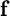with respect to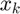at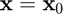using the central difference approximation with a default relative step size of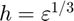, where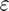is the machine zero.

pf = cpartial(f,x0,k,h) numerically evaluates the partial derivative ofwith respect toatusing the central difference approximation with a user-specified relative step size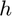.

## Input/Output Parameters

 Variable Symbol Description Format Input f multivariate, vector-valued function () 1×1function_handle x0 evaluation point n×1double k element of to differentiate with respect to 1×1double h (OPTIONAL) relative step size 1×1double Output pf partial derivative of with respect to , evaluated at m×1double

## Note

• This function requires 2 evaluations of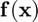.
• If the function is scalar-valued, then.

## Example #1: Partial derivative of a scalar-valued function.

Approximate the partial derivative of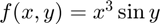with respect to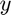at at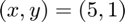using the cpartial function, and compare the result to the true result of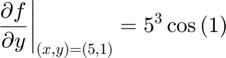First, we rewrite this function as.

f = @(x) x(1)^3*sin(x(2));


Since the second component of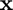represents, to approximate the derivative, we use

k = 2;


Approximating the partial derivative using the cpartial function,

pf = cpartial(f,[5;1],k)

pf =

67.5378



Calculating the error,

error = pf-5^3*cos(1)

error =

-2.2063e-09



## Example #2: Partial derivative of a vector-valued function.

Approximate the partial derivative of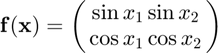with respect to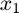at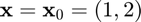using the cpartial function, and compare the result to the true result of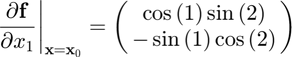Defining the function in MATLAB,

f = @(x) [sin(x(1))*sin(x(2));cos(x(1))*cos(x(2))];


Approximating the partial derivative using the cpartial function,

x0 = [1;2];             % evaluation point
k = 1;                  % element of x to differentiate with respect to
pf = cpartial(f,x0,k)   % differentiation

pf =

0.4913
0.3502



Calculating the error,

error = pf-[cos(1)*sin(2);-sin(1)*cos(2)]

error =

1.0e-11 *

-0.9040
-0.8263